### Home > A2C > Chapter Ch11 > Lesson 11.3.1 > Problem11-133

11-133.
1. Solve each equation below. Homework Help ✎

1. 2(x−1) = 64

2. 93 = 27(2x−1)

3. x6 = 29

4. 6x = 29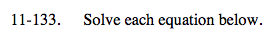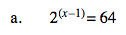Make the bases the same, and simplify.

x = 7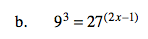See part (a).

x = 1.5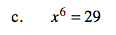$\small x=(29)^{\frac{1}{6}}$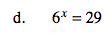log(6x) = log(29)